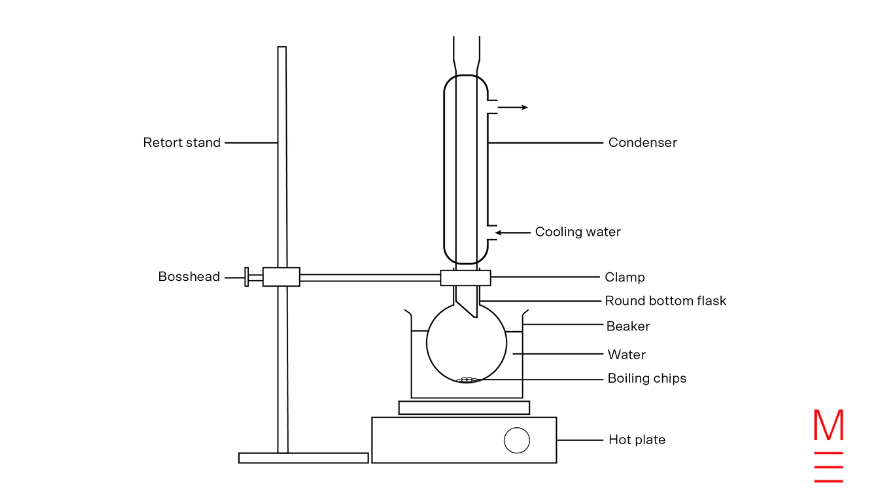# 8 Common Mistakes Chemistry Students Make in the HSC Exam

• Be careful to only give the type of response requested in the question. It is expected that students have a clear understanding of key words in questions, such as discussanalyse, and explain. These words will be used consistently in accordance with the NESA glossary of keywords.
• Use the mark allocation and the amount of space provided in the core answer book as a guide to how much you should write or draw. The space provided is more than what is needed to obtain full marks.

• Remember CORRECT RESPONSE + CONTRADICTION = 0 MARKS.
• Don’t provide multiple answers in the hope that one might be correct.
• Be careful not to provide any information that contradicts worthwhile information written earlier. A contradiction cancels out the mark you might otherwise have been awarded.
• Check that you haven’t merely reworded information given in the question for your answer.

## Mistake #3: Providing irrelevant responses

• Remember RELEVANT RESPONSE + IRRELEVANT RESPONSE = 0 MARKS
• STATEMENTS THAT DO NOT ANSWER THE QUESTION ASKED, EVEN IF THEY MAY BE RELEVANT IN ANOTHER CONTEXT, WILL NOT SCORE MARKS.

## Mistake #4: Drawing unclear, unlabelled diagrams

• It is a good idea to use a diagram where you think it clarifies an answer you are providing. Keep diagrams simple.
• Generally, it is best to draw SIMPLE TWO-DIMENSIONAL SCHEMATIC DIAGRAMS.
1. Unless specifically requested, there is usually no need to draw diagrams in three dimensions.
2. If a labelled diagram is asked for, you must label the critical parts.
3. The labelling line from a label should touch the named part and be horizontal. It should not have arrowheads unless you are indicating movement.
4. Make sure you draw the diagram and labels in pencil as mistakes can be easily rubbed out.
• An example: This diagram of reflux equipment shows a clear passage from the reflux flask to the top opening and labels are clear.Diagram of Reflux Equipment

## Mistake #5: Using incorrect chemical equations

• A chemical equation is a symbolic representation of a chemical reaction showing the reactants, products, their ratios and their states. For example:

2HCl(aq) + Na2CO3(s)  2NaCl(aq) + CO2(g) + H2O(l)

• The above equation tells us that 2 moles of aqueous hydrochloric acid react with 1 mole of solid sodium carbonate to yield 2 moles of aqueous sodium chloride, 1 mole of gaseous carbon dioxide and 1 mole of liquid water.
• For a chemical equation to achieve full marks, the equation:
a. – Must contain the correct species
b. – Must be balanced
c. – Must contain the correct states: (aq) or (l) or (s) or (g)
• To ensure the correct species are present, it is best that you memorise the major classes of reactions that occur. For example:
a. – Acid + Base  Salt + Liquid Water
b. – Acid + Metal Carbonate  Salt + Gaseous Carbon Dioxide + Liquid Water
c. –  Fuel + Oxygen  Gaseous Carbon Dioxide + Liquid Water
• Once you have written down the correct species you are then required to note their states.
• For ionic compounds, you must refer to your solubility rules.

## Mistake #6: Not knowing the solubility rules!

YEAR 12 CHEMISTRY STUDENTS ARE REQUIRED TO MEMORISE THE SOLUBILITY RULES.

• All Group 1, ammonium and nitrate compounds are soluble
• All chlorides are soluble except Ag+ and Pb2+.
• All sulfates are soluble except Ag+, Pb2+, Ca2+ and Ba2+.
• All carbonates and phosphates are insoluble except Group 1 and ammonium compounds.

## Mistake #7: Incorrectly rounding off significant figures

The final answer of all calculation questions must contain the correct number of significant figures. Remember: You are only as accurate as your least accurate value!

To calculate the number of significant figures for HSC Chemistry, use these rules:

1. Going from left to right, the first non-zero digits is significant.
2. All digits to the right are also significant.

For example, 0.300 is 3 significant figures. 103 is also 3 significant figures.

## Mistake #8: Not presenting calculations involving mole questions

• Questions involving calculations comprise of approximately 20-25% of the HSC Chemistry exam. Thus it is an area of Chemistry that must not be neglected when studying. However, one of the major causes of low marks in calculation questions is poor structure and presentation.
• Demonstration of knowledge requires appropriate structure and presentation. Therefore, to obtain a Band 6 answer, your notation is very important. The example below outlines how to notate values and how to structure a calculation question response.
• Make sure to include each step of your working to allow others to easily follow your calculation.
• It is a good idea to include units in your calculation as it can help you to spot errors in your working.
• Using NaCl as an example:
 Value Notation Mass m(NaCl) Molar mass MM(NaCl) Moles n(NaCl) Volume V(NaCl) Concentration c(NaCl)

Question:

Calculate the mass of NaCl required to form a 250.0 mL solution with a concentration of 2.00 mol L-1.